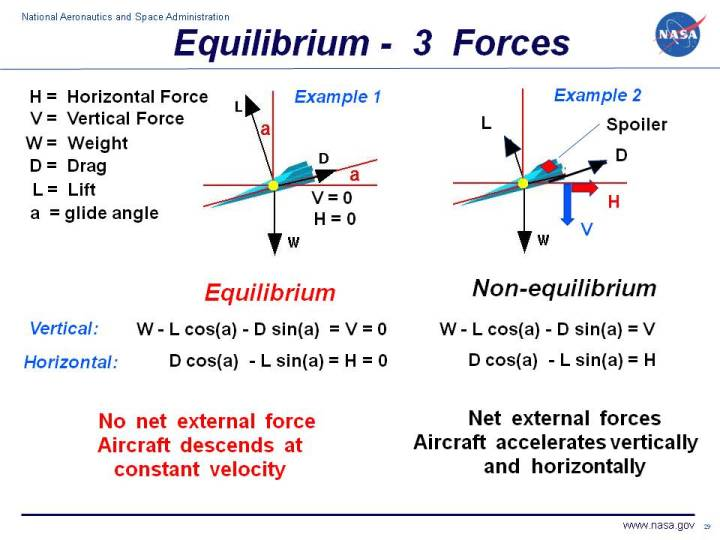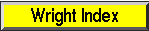+ Text Only Site
+ Non-Flash Version
+ Contact GlennA very basic concept when dealing with forces is the idea of equilibrium or balance. In general, an object can be acted on by several forces at the same time. A force is a vector quantity which means that it has both a magnitude and a direction associated with it. Two forces with the same magnitude but different directions are not equal forces. The vector sum of all of the forces acting on a body is a single force called the net force . If the net force is equal to zero, the object is said to be in equilibrium. Because there is no net force acting on an object in equilibrium, then from Newton's first law of motion, the object continues to move at a constant speed. On another page, we show the simplest example of equilibrium with two forces acting on an object. On this page, we will consider the case of a glider, which has three forces acting on it in flight. And on another page we consider the case of powered aircaft in cruise, where four forces act on the airplane. In Example 1, we show a computer drawing of a glider as it is descending. There are three forces acting on the glider; lift (L), drag (D), and weight (W). The weight is always directed towards the center of the earth, the lift is directed perpendicular to the flight path, and the drag is along the flight path. The flight path is inclined to the horizontal at an angle a. When the aircraft is in equilibrium, the vector sum of these three forces is equal to zero. Because it is a vector sum, there are two component equations, one vertical and one horizontal, which are shown below the graphic. W - L * cos(a) - D * sin(a) = V = 0 D * cos(a) - L * sin(a) = H = 0 where sin and cos are the trigonometric sine and cosine functions, V is the net vertical force, and H is the net horizontal force. Because we are looking at an equilibrium condition, H and V are equal to zero. The aircraft has a constant forward and downward velocity along the flight path. Notice that the lift, drag, and weight all continue to act on the aircraft. In equilibrium, the action of some forces are exactly balanced, or cancelled out, by other forces. In Example 2, a spoiler is deployed on the top of the glider's wing, decreasing the lift and increasing the drag. The weight remains the same. The glider is no longer in equilibrium. The equations for the forces remains the same, but there are now net horizontal and vertical forces; V and H are not equal to zero. According to Newton's second law of motion, the aircraft would begin to accelerate downward and to the right. In Example 2, the forces are not balanced and the airplane is not in equilibrium. Navigation..Beginner's Guide Home Page+ Inspector General Hotline + Equal Employment Opportunity Data Posted Pursuant to the No Fear Act + Budgets, Strategic Plans and Accountability Reports + Freedom of Information Act + The President's Management Agenda + NASA Privacy Statement, Disclaimer, and Accessibility CertificationEditor: Nancy Hall NASA Official: Nancy Hall Last Updated: May 13 2021 + Contact Glenn